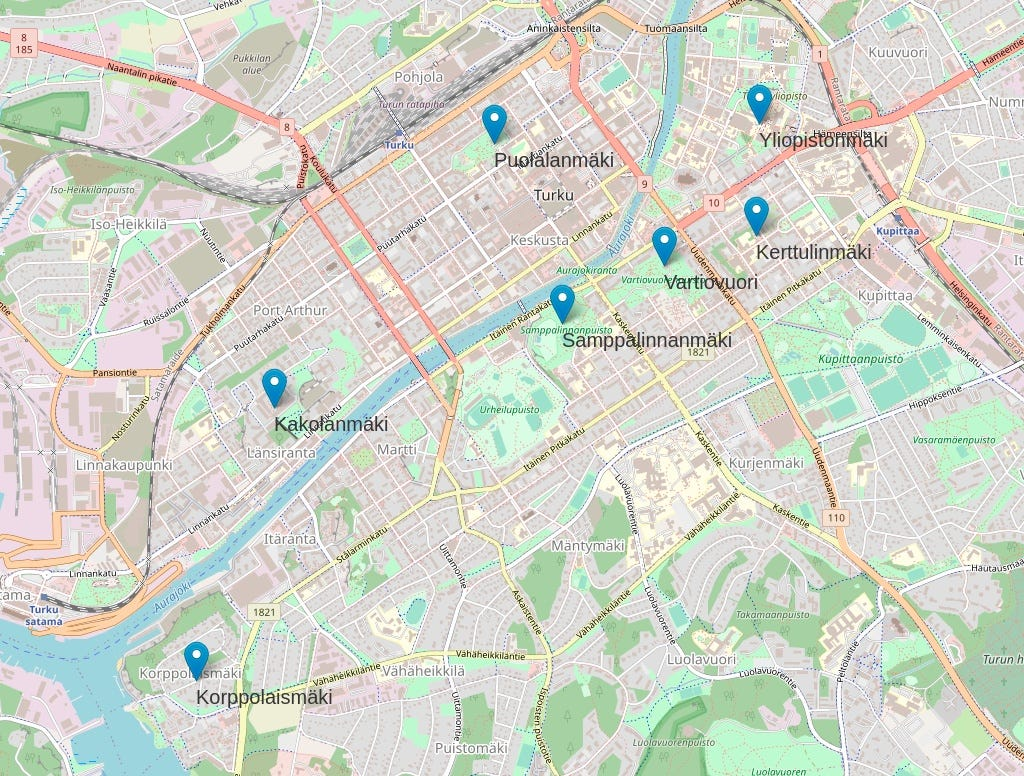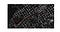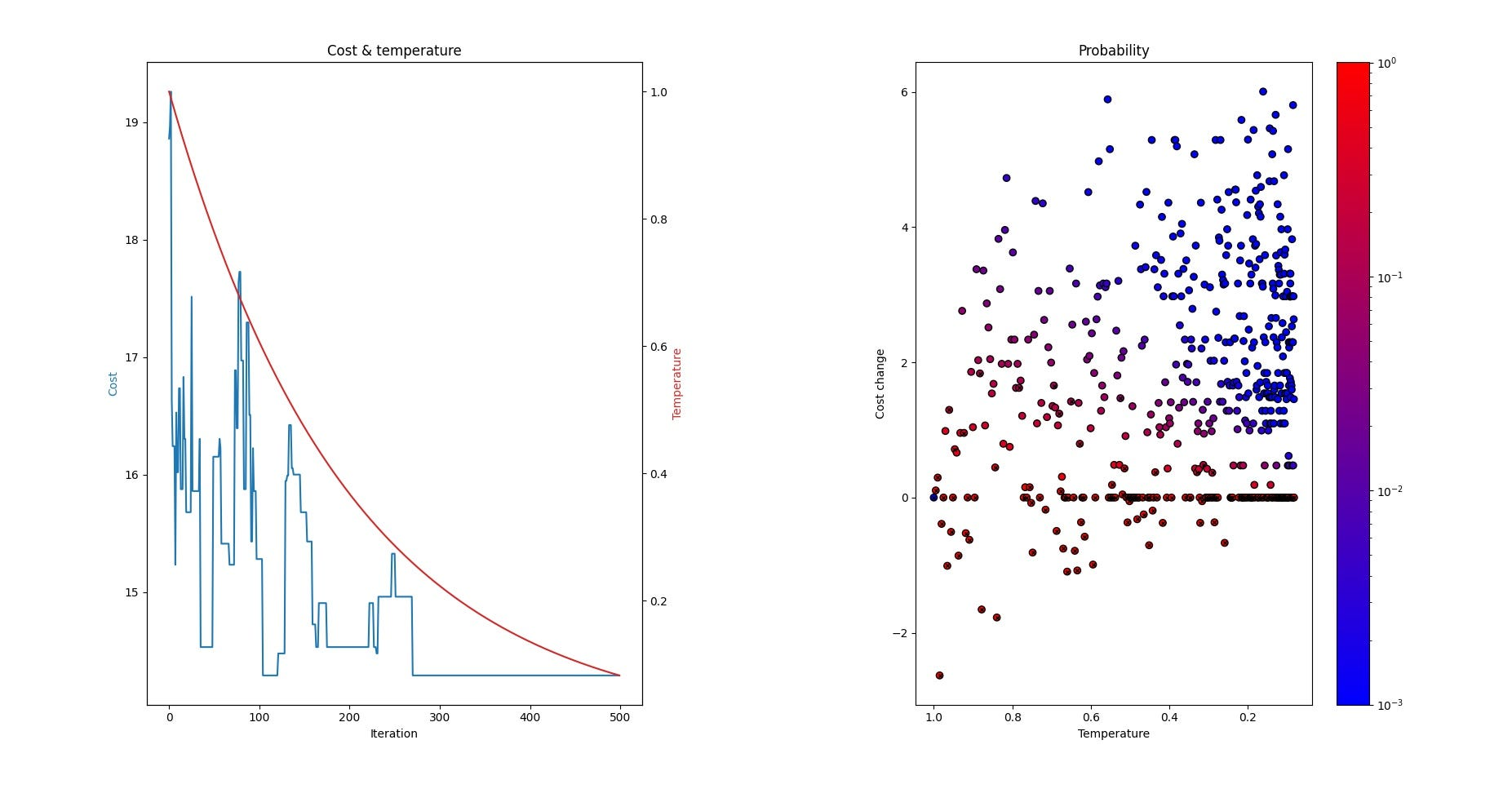# Conquering Seven Hills: Route Optimization With SA

Just like Rome has seven famous hills, we also have seven (maybe not so famous) hills in my hometown Turku. Me and my friend have a plan to arrange a walk where we visit every hill once. As we want to avoid unnecessary walking, we are faced with the classical travelling salesman problem: given the locations of the hills, we need to find the shortest route that visits each hill once and returns to the origin hill.

As there are only seven points to visit and the cost function (distance) is simple, this is an easy thing to do just by looking at the map and selecting the route manually. However, let’s try to solve this programmatically, using an algorithm called simulated annealing (SA). This kind of approach can then be used to solve similar problems that are much more complex, and it is of course much more fun like this.The seven hills. What is the shortest walking route that that visits each hill exactly once and returns to the origin hill?

Before solving the actual traveling salesman problem, we need to find a way to calculate distances between hills. Note that we need distances along roads that can be actually walked, simple bird fly distance is not enough in this case.

I was glad to find out that Python provides powerful libraries to do this. OSMnx is a Python package that lets you download spatial data from OpenStreetMap. Using this library, we can get a network of walkable roads. In more technical terms, we create a graph structure from Turku, holding information about the walkable roads. To analyze this graph, we use another library called NetworkX. It can take the graph created with OSMnx, and calculate the shortest distance between any two nodes with just one line of code. We repeat this calculation for every hill pair, and as an end result, we get matrix holding distance for every hill pair. After this, we are ready for optimization.A network of walkable roads, with the shortest distance between two hills (Vartiovuori, Kakolanmäki) marked as red as an example.

For finding the shortest route, I decided to use simulated annealing. This algorithm is especially well suited for traveling salesman type of problems as it can handle local minima well, unlike greedy algorithms that get stuck to local minima (which we have many in this kind of problem) easily.

Like so many great algorithms, also simulated annealing mimics nature’s action, physical annealing process in this case. Physical annealing is the process of heating up material and cooling it down slowly. When the temperature is high, the molecular structure of the material is weaker and is more prone to random changes. When the material cools down, the molecular structure becomes harder and is less prone to change.

Just like its physical counterpart, simulated annealing uses the concept of temperature that drives randomness in the system. The core functionality of the algorithm can be written as follows:

For every iteration:

1. Get temperature T
2. Generate mutated solution from the current solution
3. Calculate cost change, Δcost (mutated — current)
4. Replace the current solution with the mutated solution with probability P = P(T, Δcost)

5. Terminate iteration if termination conditions are met

The key ingredient of the algorithm is the fourth step where the current solution is replaced with a worse (as it has a higher cost) mutated solution with some certain probability that depends on temperature. The higher temperature, the higher is the probability and more likely it is that the current solution will be replaced with the worse solution by chance. This property makes simulated annealing so good at handling local minima: it can escape local minima as solutions with higher cost are accepted randomly. The function that produces temperature, also called schedule, is chosen so that it will mimic physical annealing: temperature is high at first and then cools down slowly when iteration proceeds.

How to get temperature, how to mutate current solution, how probability is calculated, and when to terminate iteration are all implementation details that can be tailored based on the use case. You can check my implementation from my GitHub repo.

Now that we have the matrix of distances and simulated annealing ready for use, we can just let the machine do its job.

With 500 iterations and about 10 ms time, the algorithm converges to the best possible solution, 14.3 km as the total distance. The figure below shows a typical run of the algorithm. At first comes the exploration phase: temperature is high, and the algorithm accepts some solutions that are worse than the current solution, based on calculated probability values visualized on the right figure. When iteration proceeds, the temperature cools down and the probability to accept worse solutions drops. In this stage, it is still possible that a worse solution with low Δcost is accepted as the current solution. In the end, temperature approaches zero and the algorithm works as a greedy algorithm that will accept only solutions that are better than the current one (Δcost < 0).Example run with simulated annealing. The left figure shows cost and temperature as a function of iterations. The right figure shows the probability of accepting a new mutated route (as indicated by the color bar) as the current solution, as a function of temperature and cost change.

And finally, here is the optimized route visualized on the map:Optimized walking route through the hills (0 -> 1 -> 2 -> … -> 6 -> 0), with 14.3 km total distance.